Math resources Geometry Area

Area of a trapezoid

# Area of a trapezoid

Here you will learn about finding the area of a trapezoid, including how to decompose a trapezoid into other shapes, finding missing dimensions, and applying this to real world scenarios.

Students first learn about the area of trapezoids in 6 th grade with their work in geometry. They expand upon their knowledge as they progress through middle and high school.

## What is the area of a trapezoid?

The area of a trapezoid is the amount of space inside the trapezoid. The area is measured in units squared \left(\mathrm{cm}^2, \mathrm{~m}^2, i \mathrm{n}^2, f t^2, e t c . \right)

A trapezoid (also known as a trapezium) is a quadrilateral with exactly one pair of parallel sides. The parallel sides are referred to as the bases of the trapezoid and are indicated using small arrows.

An isosceles trapezoid is a trapezoid where the base angles and legs are equal.

To find the area of a trapezoid:

Strategy 1: Strategy 2:

Decompose the trapezoid
into a rectangle and a triangle.

Use the formula for the area of a trapezoid.

\text {Area of trapezoid}=\cfrac{1}{2} \, \times h \times(\text {base } 1+\text {base } 2)

The parallel sides are the bases, where one base is
the shorter base and the other is the longer base.

Area of the rectangle:

\begin{aligned} & A=\text { base } \times \text { height } \\\\ & \text { height }=5 \mathrm{~cm} \\ & \text { base }=6 \mathrm{~cm} \\\\ & A=6 \times 5 \\ & A=30 \mathrm{~cm}^2 \end{aligned}

Area of the triangle:

\begin{aligned} & A=\cfrac{1}{2} \, \times \text { base } \times \text { height } \\\\ & \text { base }=4 \\ & \text { height }=5 \\\\ & A=\cfrac{1}{2} \, \times 4 \times 5 \\\\ & A=10 \mathrm{~cm}^2 \end{aligned}

To find the area of the trapezoid, add
the areas of the rectangle and the
triangle together.

\begin{aligned} & \text { Area of trapezoid }=30+10 \\ & \text { Area of trapezoid }=40 \mathrm{~cm}^2 \end{aligned}

\begin{aligned} & \text { height }=5 \\ & \text { base } 1=6 \\ & \text { base } 2=10 \\\\ & \text { Area }=\cfrac{1}{2} \, \times 5 \times(6+10) \rightarrow \text {sum of the bases} \\\\ & \text { Area }=\cfrac{1}{2} \, \times 5 \times(16) \\\\ & \text { Area }=40 \mathrm{~cm}^2 \end{aligned}

### What is the area of a trapezoid?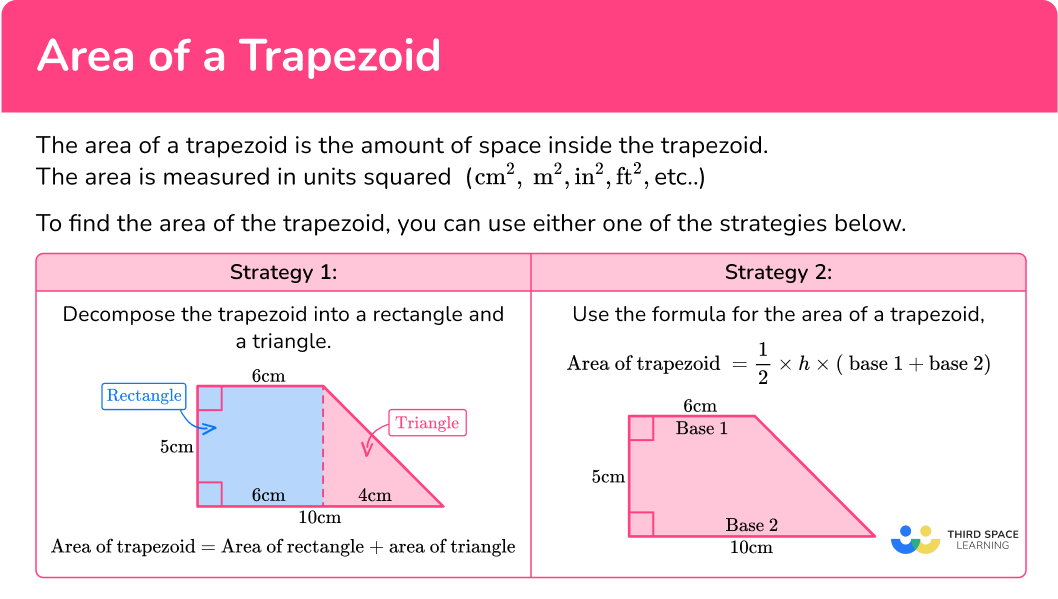## Common Core State Standards

How does this relate to 6 th and 7 th grade math?

• Grade 6 – Geometry (6.G.A.1)
Find the area of right triangles, other triangles, special quadrilaterals, and polygons by composing into rectangles or decomposing into triangles and other shapes; apply these techniques in the context of solving real-world and mathematical problems.

• Grade 7 – Geometry (7.G.B.6)
Solve real-world and mathematical problems involving area, volume and surface area of two- and three-dimensional objects composed of triangles, quadrilaterals, polygons, cubes, and right prisms.

## How to find the area of a trapezoid

In order to find the area of a trapezoid:

1. Identify the given dimensions.
2. Substitute the given values into the formula.

## Area of a trapezoid examples

### Example 1: area of trapezoid by decomposing into other shapes

Find the area of the trapezoid below:

1. Identify the given dimensions.

Decompose the trapezoid into a rectangle and a triangle.

Rectangle dimensions:

\begin{aligned}& \text { height }=6 \mathrm{~cm} \\\\ & \text { base }=3 \mathrm{~cm}\end{aligned}

Triangle dimensions:

\text { height }=6 \mathrm{~cm}

To find the base of the triangle, subtract, 13-3 =10.

\text { base }=10 \mathrm{~cm}

2Substitute the given values into the formula.

\begin{aligned} & \text { Area of rectangle }=base \times height \\\\ & \text { Area of rectangle }=3 \times 6 \\\\ & \text { Area of rectangle }=18 \end{aligned}

\begin{aligned} & \text {Area of triangle }=\cfrac{1}{2} \times base \times height \\\\ & \text {Area of triangle }=\cfrac{1}{2} \times 10 \times 6 \\\\ & \text {Area of triangle }=30 \end{aligned}

To find the area of the trapezoid, add the area of the rectangle and the area of the triangle.

\begin{aligned} & \text { Area of trapezoid }=\text { Area of rectangle }+\text { Area of triangle } \\\\ & \text { Area of trapezoid }=18+30 \\\\ & \text { Area of trapezoid }=48 \end{aligned}

The area of the trapezoid is 48 \mathrm{~cm}^2.

### Example 2: area of an isosceles trapezoid by decomposing into other shapes

Find the area of the isosceles trapezoid.

Decompose the isosceles trapezoid into a rectangle and two equal triangles.

Triangle dimensions (both triangles are equal (congruent)).

\text { height }=38 \mathrm{~m}

To find the base of one of the triangles, subtract 20 from 34.

34-20=14

Since both the triangles are equal, divide 14 by 2.

14 \div 2=7

The base of each triangle is 7 \mathrm{~m}.

\text { base }=7 \mathrm{~m}

Rectangle dimensions:

\begin{aligned}& \text { height }=38 \mathrm{~m} \\\\ & \text { base }=20 \mathrm{~m}\end{aligned}

Triangle:

\begin{aligned}& \text { Area }=\cfrac{1}{2} \times \text { base } \times \text { height } \\\\ & \text { Area }=\cfrac{1}{2} \times 7 \times 38 \\\\ & \text { Area }=133\end{aligned}

Since there are two triangles that are equal, the total area of both triangles is:

2 \times 133=266

Rectangle

\begin{aligned}& \text { Area }=\text { base } \times \text { height } \\\\ & \text { Area }=20 \times 38 \\\\ & \text { Area }=760 \\\\\\ & \text { Area of Trapezoid }=\text {Area of two equal triangles }+ \text {Area of rectangle } \\\\ & \text { Area of Trapezoid }=266+760 \\\\ & \text { Area of Trapezoid }=1026 \end{aligned}

The area of the trapezoid is 1026 \mathrm{~m}^2.

### Example 3: area of trapezoid changing the units

Find the area of the trapezoid.

First change 950 \, cm to meters.

950 \mathrm{~cm}=9.50 \text { meters }

The trapezoid can be decomposed into one rectangle and one triangle.

The dimensions of the rectangle are:

\begin{aligned}& \text { base }=9.5 \mathrm{~m} \\\\ & \text { height }=8 \mathrm{~m}\end{aligned}

The dimensions of the triangle are:

\begin{aligned}& \text { height }=8 \mathrm{~m} \\\\ & \text { base }=14 \mathrm{~m}-9.5 \mathrm{~m} \\\\ & \text { base }=4.5 \mathrm{~m}\end{aligned}

\begin{aligned}& \text { Area of rectangle }=\text { base } \times \text { height } \\\\ & \text { Area of rectangle }=9.5 \times 8 \\\\ & \text { Area of rectangle }=76 \\\\\\ & \text { Area of triangle }=\cfrac{1}{2} \times \text { base } \times \text { height } \\\\ & \text { Area of triangle }=\cfrac{1}{2} \times 4.5 \times 8 \\\\ & \text { Area of triangle }=18 \\\\\\ & \text { Area of trapezoid }=\text { Area of rectangle }+ \text { Area of triangle } \\\\ & \text { Area of trapezoid }=76+18 \\\\ & \text { Area of trapezoid }=94 \end{aligned}

The area of the trapezoid is 94 \mathrm{~m}^2.

### Example 4: finding area of a trapezoid formula

Use the formula for area of a trapezoid, A=\cfrac{1}{2} \times h \times(\text { base } 1+\text { base } 2), to find the area of the trapezoid.

The parallel sides of the trapezoids are the bases.

\begin{aligned}& \text { base } 1=13 \mathrm{~in} \\\\ & \text { base } 2=17 \mathrm{~in} \end{aligned}

The height of the trapezoid is the perpendicular distance between the bases (forms a right angle).

\text { height }=22 \mathrm{~in}

\begin{aligned}& \text { Area of trapezoid }=\cfrac{1}{2} \times h \times(\text { base } 1+\text { base } 2) \\\\ & \text { Area of trapezoid }=\cfrac{1}{2} \times 22 \times(13+17) \\\\ & \text { Area of trapezoid }=330\end{aligned}

The area of the trapezoid is 330 \mathrm{~in}^2.

### Example 5: find missing dimension

Find the height of the trapezoid if the area is 540 \mathrm{~cm}^2.

\begin{aligned}& \text { Area of trapezoid }=540 \mathrm{~cm}^2 \\\\ & \text { base } 1=32 \mathrm{~cm} \\\\ & \text { base } 2=40 \mathrm{~cm} \end{aligned}

Use the formula for the area of the trapezoid to find the height.

\begin{aligned} &\text { Area of trapezoid }=\cfrac{1}{2} \times \text { height } \times(\text { base } 1+\text { base } 2) \\\\ & 540=\cfrac{1}{2} \times h \times(32+40) \\\\ & 540=\cfrac{1}{2} \times h \times 72 \\\\ & 540=36 \times h \\\\ & 540 \div 36=36 \times h\div 36 \\\\ & 15=h\end{aligned}

The height of the trapezoid is 15 \mathrm{~cm}.

### Example 6: word problem

Sarah is looking to design the flower bed below at the front of her house in the shape of a trapezoid. She would like to cover the entire flower bed with rose bushes. Each bush needs 1.5 \mathrm{~m}^2 to allow the roots to spread out evenly.

What is the maximum number of rose bushes she can plant on her flower bed? Use the formula, \text { Area }=\cfrac{1}{2} \times \text { height } \times(\text { base } 1+\text { base } 2).

\begin{aligned}& \text { base } 1=4.5 \mathrm{~m} \\\\ & \text { base } 2=1.5 \mathrm{~m} \\\\ & \text { height }=3 \mathrm{~m} \end{aligned}

Rose bush needs 1.5 \mathrm{~m}^2.

\begin{aligned}& \text { Area of trapezoid }=\cfrac{1}{2} \times 3 \times(4.5+1.5) \\\\ & \text { Area of trapezoid }=9 \end{aligned}

The area of the trapezoid flower bed is 9 \mathrm{~m}^2. Since each rose bush needs 1.5 \mathrm{~m}^2 in space, divide 9 by 1.5.

9 \div 1.5=6

A maximum of 6 rose bushes can be planted.

### Teaching tips for area of trapezoid

• Provide students with investigative activities that allow them to informally derive the formula for the area of a trapezoid.

• Use digital platforms such as Desmos so students can investigate strategies to find the area of a trapezoid.

• Although worksheets provide an opportunity for students to practice skills, using digital platforms that focus on game play is more effective in engaging students.

### Easy mistakes to make

• Using incorrect units for the answer
Area is a square unit. After calculating the area of a trapezoid, be sure to square the dimension. For example, if using the formula for the area of a trapezoid to find the area of the trapezoid.

\begin{aligned}& A=\cfrac{1}{2} \times 7 \mathrm{~cm} \times(4 \mathrm{~cm}+9 \mathrm{~cm}) \\\\ & A=\cfrac{1}{2} \times 7 \mathrm{~cm} \times(13 \mathrm{~cm}) \\\\ & A=45.5 \mathrm{~cm}^2\end{aligned}

• Forgetting to convert measures to a common unit
Before calculating the area of a trapezoid or any 2D figure, make sure all units are the same. For example, if a base length is in meters and the height is in centimeters, be sure to change the dimensions all to meters or all to centimeters.

• Using length of the non-parallel sides when calculating area and not the height
The height of the trapezoid is always perpendicular (forms a right angle) to both bases.

The height of this trapezoid is 7 \mathrm{~cm} not 8 \mathrm{~cm}.

### Practice area of a trapezoid questions

1. If you decompose the isosceles trapezoid below into a rectangle and two congruent triangles, what would the base length of one of the triangles be?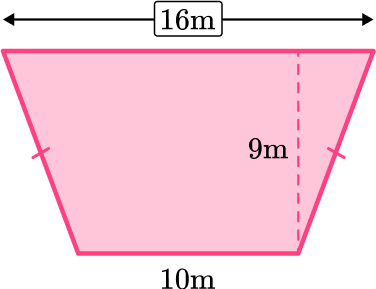9 \, m6 \, m10 \, m3 \, m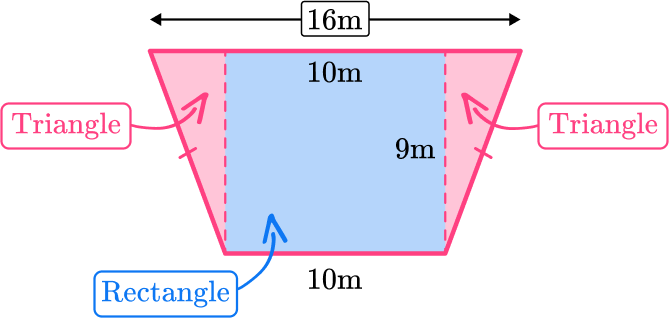16-10=6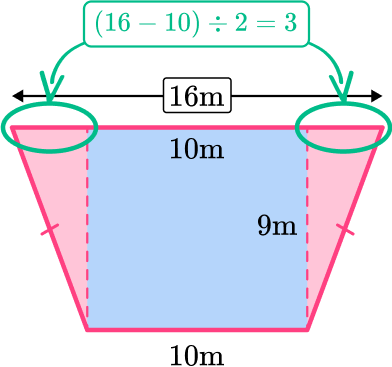The lengths of the bases circled in red sum to 6 \, m.

So the length of one base is half of 6.

6 \div 2=3

The length of one base is 3 \, m.

2. Find the area of the trapezoid.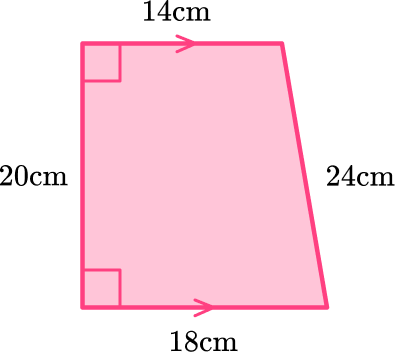384 \mathrm{~cm}^2384 \mathrm{~cm}320 \mathrm{~cm}^2320 \mathrm{~cm}Decompose the trapezoid into a rectangle and a triangle.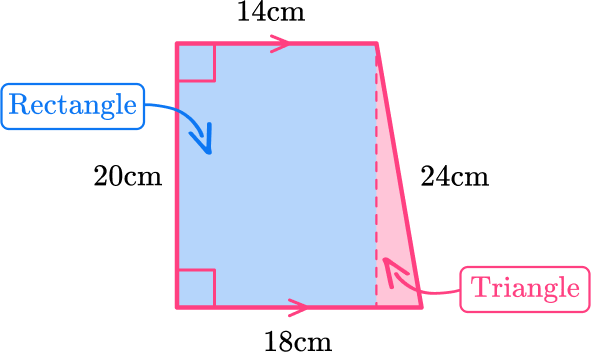The area of the rectangle is:

\begin{aligned}& A=b \times h \\\\ & \text { base }=14 \\\\ & \text { height }=20 \\\\ & A=14 \times 20 \\\\ & A=280 \mathrm{~cm}^2\end{aligned}

The area of the triangle is:

\begin{aligned}& A=\cfrac{1}{2} \times b \times h \\\\ & \text { base }=4 \\\\ & \text { height }=20\end{aligned}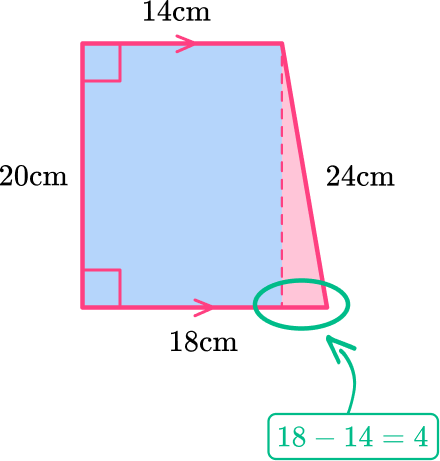\begin{aligned}& A=\cfrac{1}{2} \times 4 \times 20 \\\\ & A=40 \mathrm{~cm}^2\end{aligned}

To find the area of the trapezoid, add the area of the rectangle and the area of the triangle, 40+280=320.

The area of the trapezoid is 320 \mathrm{~cm}^2.

OR

You can use the formula for the area of a trapezoid.

\begin{aligned}& \text { Area of trapezoid }=\cfrac{1}{2} \times h \times(\text { base } 1+\text { base } 2) \\\\ & \text { height }=20 \\\\ & \text { base } 1=14 \\\\ & \text { base } 2=18 \\\\ & \text { Area of trapezoid }=\cfrac{1}{2} \times 20 \times(14+18) \\\\ & \text { Area of trapezoid }=10 \times 32 \\\\ & \text { Area of trapezoid }=320 \mathrm{~cm}^2\end{aligned}

3. Find the area of the isosceles trapezoid below: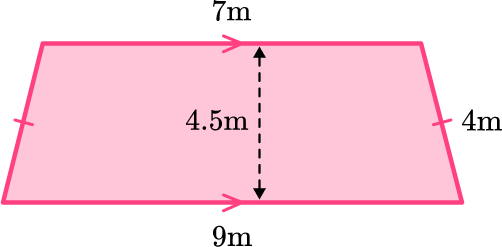36 \mathrm{~m}^232 \mathrm{~m}^267.7 \mathrm{~m}^220.5 \mathrm{~m}^2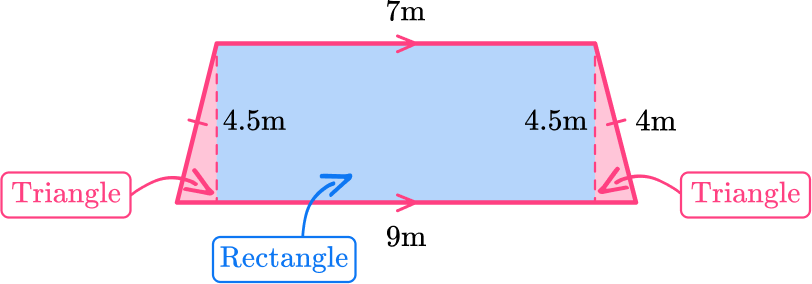Decompose the trapezoid into one rectangle with dimensions, \text { base }=7 and the \text { height }=4.5.

The area of the rectangle is:

\begin{aligned} A=b \times h \\\\ \text { base }=7 \end{aligned}

\begin{aligned}& \text { height }=4.5 \\\\ & A=7 \times 4.5 \\\\ & A=31.5 \end{aligned}

The two triangles are congruent, where the height of the triangles is 4.5 and the base of one of the triangles can be found by: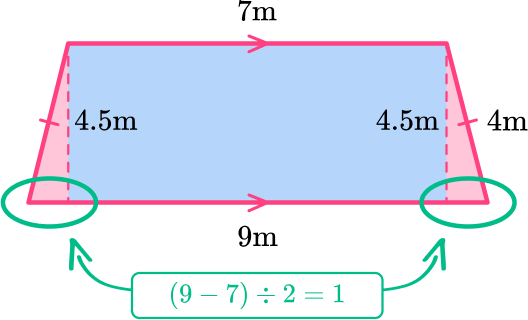The area of a triangle:

\begin{aligned}& \text { base }=1 \\\\ & \text { height }=4.5 \\\\ & A=\cfrac{1}{2} \times 4.5 \times 1 \\\\ & A=2.25\end{aligned}

Both triangles are equal so the area of the two triangles is, 2 \times 2.25=4.5

To find the area of the trapezoid, add the area of the rectangle and the two triangles.

\begin{aligned}& A=31.5+4.5 \\\\ & A=36 \mathrm{~m}^2\end{aligned}

OR

You can use the formula for the area of a trapezoid.

\begin{aligned}& \text { Area of trapezoid }=\cfrac{1}{2} \times h \times(\text { base } 1+\text { base } 2) \\\\ & \text { height }=4.5 \\\\ & \text { base } 1=7 \\\\ & \text { base } 2=9 \\\\ & \text { Area of trapezoid }=\cfrac{1}{2} \times 4.5 \times(7+9) \\\\ & \text { Area of trapezoid }=2.25 \times 16 \\\\ & \text { Area of trapezoid }=36 \mathrm{~m}^2\end{aligned}

4. Find the area of the trapezoid below: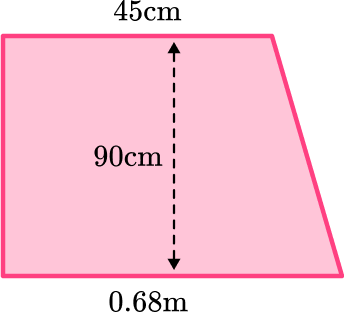5085 \mathrm{~cm}^22055.6 \mathrm{~m}^22055.6 \mathrm{~cm}^25085 \mathrm{~m}^2First convert base 2 into centimeters by multiplying 0.68 \times 100=68.

0.68 \mathrm{~cm}=68 \mathrm{~m}

You can decompose the trapezoid into a triangle and a rectangle to find the area (see below) or use the formula for finding the area of a trapezoid,

\text { A }=\cfrac{1}{2} \times h \times(\text { base } 1+\text { base } 2)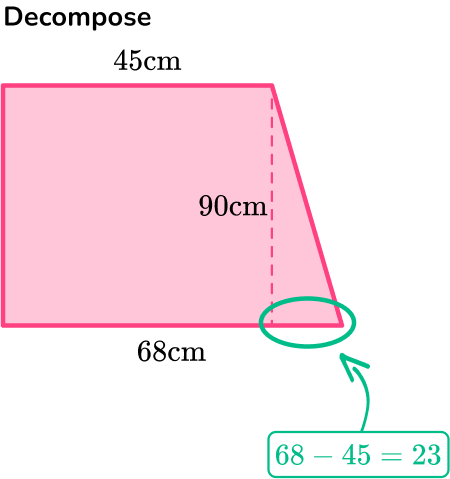Area of rectangle:

\begin{aligned}& A=b \times h \\\\ & \text { base }=45 \\\\ & \text { height }=90 \\\\ & A=45 \times 90 \\\\ & A=4050\end{aligned}

Area of triangle:

\begin{aligned}& A=\cfrac{1}{2} \times b \times h \\\\ & \text { base }=23 \\\\ & \text { height }=90 \\\\ & A=\cfrac{1}{2} \times 23 \times 90 \\\\ & A=1035\end{aligned}

\begin{aligned}& \text { Area of trapezoid }=\text { Area of rectangle }+ \text { Area of triangle } \\\\ & \text { Area of trapezoid }=4050+1035 \\\\ & \text { Area of trapezoid }=5085 \mathrm{~cm}^2\end{aligned}

OR

\begin{aligned}& \text { Area of trapezoid }=\cfrac{1}{2} \times h \times(\text { base } 1+\text { base } 2) \\\\ & \text { height }=90 \\\\ & \text { base } 1=45 \\\\ & \text { base } 2=68 \\\\ & \text { Area of trapezoid }=\cfrac{1}{2} \times 90 \times(45+68) \\\\ & \text { Area of trapezoid }=45 \times 113 \\\\ & \text { Area of trapezoid }=5085 \mathrm{~cm}^2\end{aligned}

5. Find the area of the trapezoid.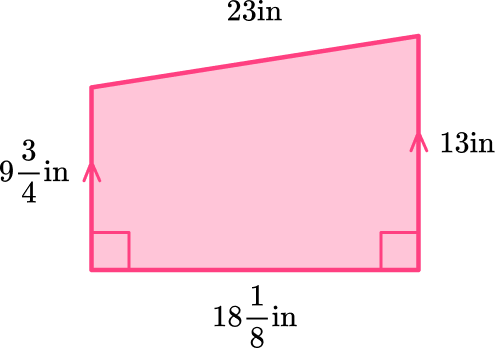261 \cfrac{5}{8} \mathrm{~in}^2206 \cfrac{11}{64} \mathrm{~in}206 \cfrac{11}{64} \text { in}^2261 \cfrac{5}{8} \text { in }You can decompose the trapezoid into a rectangle and a triangle to find the area, or you can use the formula for the area of a trapezoid.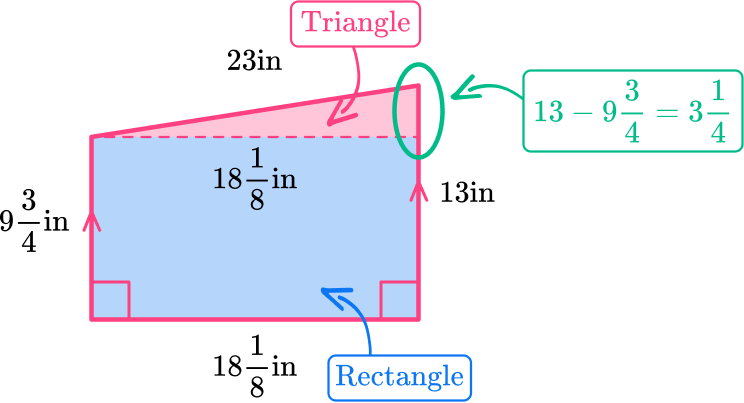Area of rectangle:

\begin{aligned}& A=b \times h \\\\ & \text { base }=18 \cfrac{1}{8} \\\\ & \text { height }=9 \cfrac{3}{4} \\\\ & A=18 \cfrac{1}{8} \times 9 \cfrac{3}{4} \\\\ & A=\cfrac{145}{8} \times \cfrac{39}{4} \\\\ & A=\cfrac{5655}{32} \\\\ & A=176 \cfrac{23}{32}\end{aligned}

Area of triangle:

\begin{aligned}& A=\cfrac{1}{2} \times b \times h \\\\ & \text { base }=18 \cfrac{1}{8} \\\\ & \text { height }=3 \cfrac{1}{4} \\\\ & A=\cfrac{1}{2} \times 18 \cfrac{1}{8} \times 3 \cfrac{1}{4} \\\\ & A=\cfrac{1}{2} \times \cfrac{145}{8} \times \cfrac{13}{4} \\\\ & A=\cfrac{1885}{64} \\\\ & A=29 \cfrac{29}{64}\end{aligned}

\begin{aligned}& \text { Area of trapezoid }=\text { Area of rectangle }+ \text { Area of triangle } \\\\ & \text { Area of trapezoid }=176 \cfrac{23}{32}+29 \cfrac{29}{64} \\\\ & \text { Area of trapezoid }=176 \cfrac{46}{64}+29 \cfrac{29}{64} \\\\ & \text { Area of trapezoid }=205 \cfrac{75}{64}=206 \cfrac{11}{64} \mathrm{~in}^2\end{aligned}

OR

\begin{aligned}& \text { Area of trapezoid }=\cfrac{1}{2} \times h \times(\text { base } 1+\text { base } 2) \\\\ & \text { height }=18 \cfrac{1}{8} \\\\ & \text { base } 1=9 \cfrac{3}{4} \\\\ & \text { base } 2=13 \\\\ & A=\cfrac{1}{2} \times 18 \cfrac{1}{8} \times\left(9 \cfrac{3}{4}+13\right) \\\\ & A=\cfrac{1}{2} \times 18 \cfrac{1}{8} \times 22 \cfrac{3}{4} \\\\ & A=\cfrac{1}{2} \times \cfrac{145}{8} \times \cfrac{91}{4} \\\\ & A=\cfrac{13195}{64}=206 \cfrac{11}{64} \mathrm{~in}^2\end{aligned}

6. Find the height of a trapezoid if the area is 54 \mathrm{~m}^2.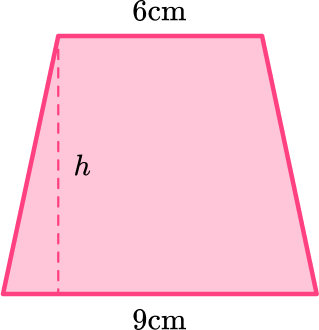7.2 \mathrm{~cm}7.2 \mathrm{~cm}^27.5 \mathrm{~cm}7.5 \mathrm{~cm}^2The formula for the area of a trapezoid is:

\begin{aligned}& \text { Area }=\cfrac{1}{2} \times h \times(\text { base } 1+\text { base } 2) \\\\ & \text { Area }=54 \\\\ & \text { base } 1=6 \\\\ & \text { base } 2=9 \\\\ & 54=\cfrac{1}{2} \times h \times(6+9) \\\\ & 54=\cfrac{1}{2} \times h \times 15 \\\\ & 54=7.5 \times h \\\\ & 54 \div 7.5=7.5  \times h\div 7.5 \\\\ & 7.2=h\end{aligned}

The height of the trapezoid is 7.2 \, cm.

7. Sally is looking to design the garden below, at the back of her house. She would like to cover the entire garden with grass. An individual carpet of grass covers an area of 4 square meters. How many rolls of grass will be needed to cover the entire garden?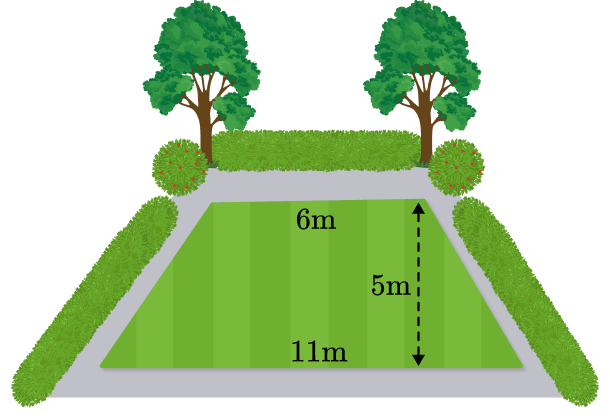10 \text { rolls of grass }42 \text { rolls of grass }11 \text { rolls of grass }12 \text { rolls of grass }\begin{aligned} & \text { Area of trapezoid }=\cfrac{1}{2} \times h \times(\text { base } 1+\text { base } 2) \\\\ &\text { Area of trapezoid }=\cfrac{1}{2} \times 5 \times(6+11) \\\\ & \text { Area of trapezoid }=\cfrac{1}{2} \times 5 \times 17 \\\\ & \text { Area of trapezoid }=42.5 \mathrm{~m}^2\end{aligned}

The area of the garden is 42.5 \, m^2 and an individual roll of grass covers 4 \mathrm{~m}^2. So to find the total number of rolls of grass needed, divide the area by the area of one roll.

42.5 \div 4=10.625

Since Sally cannot buy 0.625 of a roll of grass, she will need to purchase 11 rolls of grass.

## Area of trapezoid FAQs

Do you need to use the Pythagorean Theorem to find the area of a trapezoid?

When you get into high school, you will explore area of trapezoids in greater depth, where you will have to apply the Pythagorean Theorem to find a missing line segment of the trapezoid. You will also use the Pythagorean Theorem to find the perimeter of a trapezoid.

## Still stuck?

At Third Space Learning, we specialize in helping teachers and school leaders to provide personalized math support for more of their students through high-quality, online one-on-one math tutoring delivered by subject experts.

Each week, our tutors support thousands of students who are at risk of not meeting their grade-level expectations, and help accelerate their progress and boost their confidence.